# Oporowski, Bogdan

## Book Thickness of Subdivisions ★★

Author(s): Blankenship; Oporowski

Letbe a finite undirected simple graph.

A-page book embedding ofconsists of a linear orderofand a (non-proper)-colouring of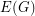such that edges with the same colour do not cross with respect to. That is, if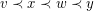for some edges, thenandreceive distinct colours.

One can think that the vertices are placed along the spine of a book, and the edges are drawn without crossings on the pages of the book.

The book thickness of, denoted by btis the minimum integerfor which there is a-page book embedding of.

Letbe the graph obtained by subdividing each edge ofexactly once.

Conjecture   There is a functionsuch that for every graph,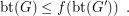Keywords: book embedding; book thickness

## 5-coloring graphs with small crossing & clique numbers ★★

Author(s): Oporowski; Zhao

For a graph, we letdenote the crossing number of, and we letdenote the size of the largest complete subgraph of.

Question   Does every graphwithandhave a 5-coloring?

Keywords: coloring; crossing number; planar graph

## Bounded colorings for planar graphs ★★

Author(s): Alon; Ding; Oporowski; Vertigan

Question   Does there exists a fixed functionso that every planar graph of maximum degreehas a partition of its vertex set into at most three setsso that for, every component of the graph induced by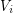has size at most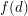?

Keywords: coloring; partition; planar graph